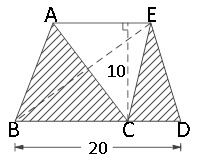### Sample Problem

What is the area of the shaded part?#### Solution

The sum ofis their base times the height which is 10 for both of the triangles, and then divided by two. We have (BC × 10 + CD × 10) ÷ 2, which equals ( (BC + CD) × 10) ÷ 2. Since we see that BC + CD is 20, we actually have 20 × 10 ÷ 2, which equals 100.